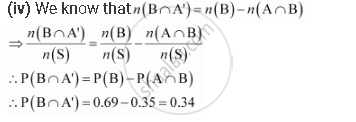# A and B Are Two Events Such that P(A) = 0.54, P(B) = 0.69 and P(A ∩ B) = 0.35. - Mathematics

A and B are two events such that P(A) = 0.54, P(B) = 0.69 and P(A ∩ B) = 0.35.

Find

(i) P(A ∩ B)

(ii) P(A′ ∩ B′)

(iii) P(A ∩ B′) (iv) P(B ∩ A′)

#### Solution

It is given that P(A) = 0.54, P(B) = 0.69, P(A ∩ B) = 0.35

(i) We know that P (A ∪ B) = P(A) + P(B) − P(A ∩ B)

∴P (A ∪ B) = 0.54 + 0.69 − 0.35 = 0.88

(ii) A′ ∩ B′ = (A ∪ B)′ [by De Morgan’s law]

∴P(A′ ∩ B′) = P(A ∪ B)′ = 1 − P(A ∪ B) = 1 − 0.88 = 0.12

(iii) P(A ∩ B′) = P(A) − P(A ∩ B)

= 0.54 − 0.35

= 0.19Concept: Event - Algebra of Events
Is there an error in this question or solution?

#### APPEARS IN

NCERT Class 11 Mathematics
Chapter 16 Probability
Q 7 | Page 409